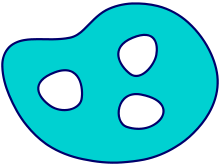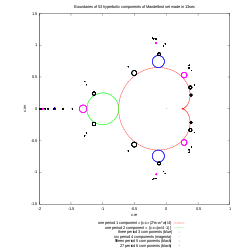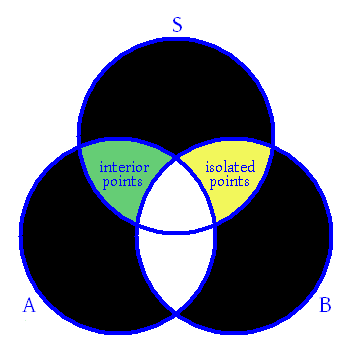Boundary (topology)A set (in light blue) and its boundary (in dark blue).

In topology and mathematics in general, the boundary of a subset S of a topological space X is the set of points which can be approached both from S and from the outside of S. More precisely, it is the set of points in the closure of S, not belonging to the interior of S. An element of the boundary of S is called a boundary point of S. The term boundary operation refers to finding or taking the boundary of a set. Notations used for boundary of a set S include bd(S), fr(S), and S. Some authors (for example Willard, in General Topology) use the term frontier instead of boundary in an attempt to avoid confusion with the concept of boundary used in algebraic topology and manifold theory. However, frontier sometimes refers to a different set, which is the set of boundary points which are not actually in the set; that is, S \ S.

A connected component of the boundary of S is called a boundary component of S.

If the set consists of discrete points only, then the set has only a boundary and no interior.

Common definitions

There are several common (and equivalent) definitions to the boundary of a subset S of a topological space X:

• the closure of S without the interior of S: ∂S = S \ So.
• the intersection of the closure of S with the closure of its complement: ∂S = S  (X \ S).
• the set of points p of X such that every neighborhood of p contains at least one point of S and at least one point not of S.

ExamplesBoundary of hyperbolic components of Mandelbrot set

Consider the real line R with the usual topology (i.e. the topology whose basis sets are open intervals). One has

• ∂(0,5) = ∂[0,5) = ∂(0,5] = ∂[0,5] = {0,5}
• ∂∅ =
• Q = R
• ∂(Q  [0,1]) = [0,1]

These last two examples illustrate the fact that the boundary of a dense set with empty interior is its closure.

In the space of rational numbers with the usual topology (the subspace topology of R), the boundary of, where a is irrational, is empty.

The boundary of a set is a topological notion and may change if one changes the topology. For example, given the usual topology on R2, the boundary of a closed disk Ω = {(x,y) | x2 + y2  1} is the disk's surrounding circle: ∂Ω = {(x,y) | x2 + y2 = 1}. If the disk is viewed as a set in R3 with its own usual topology, i.e. Ω = {(x,y,0) | x2 + y2  1}, then the boundary of the disk is the disk itself: ∂Ω = Ω. If the disk is viewed as its own topological space (with the subspace topology of R2), then the boundary of the disk is empty.

Properties

• The boundary of a set is closed.
• The boundary of the interior of a set as well as the boundary of the closure of a set are both contained in the boundary of the set.
• A set is the boundary of some open set if and only if it is closed and nowhere dense.
• The boundary of a set is the boundary of the complement of the set: ∂S = ∂(SC).
• The interior of the boundary of a closed set is the empty set.

Hence:

• p is a boundary point of a set if and only if every neighborhood of p contains at least one point in the set and at least one point not in the set.
• A set is closed if and only if it contains its boundary, and open if and only if it is disjoint from its boundary.
• The closure of a set equals the union of the set with its boundary. S = S  S.
• The boundary of a set is empty if and only if the set is both closed and open (that is, a clopen set).
• The interior of the boundary of the closure of a set is the empty set.Conceptual Venn diagram showing the relationships among different points of a subset S of Rn. A = set of limit points of S, B = set of boundary points of S, area shaded green = set of interior points of S, area shaded yellow = set of isolated points of S, areas shaded black = empty sets. Every point of S is either an interior point or a boundary point. Also, every point of S is either an accumulation point or an isolated point. Likewise, every boundary point of S is either an accumulation point or an isolated point. Isolated points are always boundary points.

Boundary of a boundary

For any set S, ∂S ⊇ ∂∂S, with equality holding if and only if the boundary of S has no interior points, which will be the case for example if S is either closed or open. Since the boundary of a set is closed, ∂∂S = ∂∂∂S for any set S. The boundary operator thus satisfies a weakened kind of idempotence.

In discussing boundaries of manifolds or simplexes and their simplicial complexes, one often meets the assertion that the boundary of the boundary is always empty. Indeed, the construction of the singular homology rests critically on this fact. The explanation for the apparent incongruity is that the topological boundary (the subject of this article) is a slightly different concept from the boundary of a manifold or of a simplicial complex. For example, the boundary of an open disk viewed as a manifold is empty, while its boundary in the sense of topological space is the circle surrounding the disk.